Question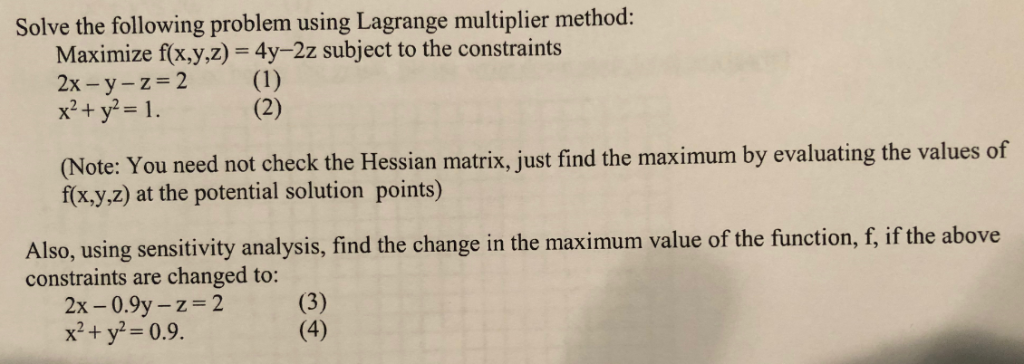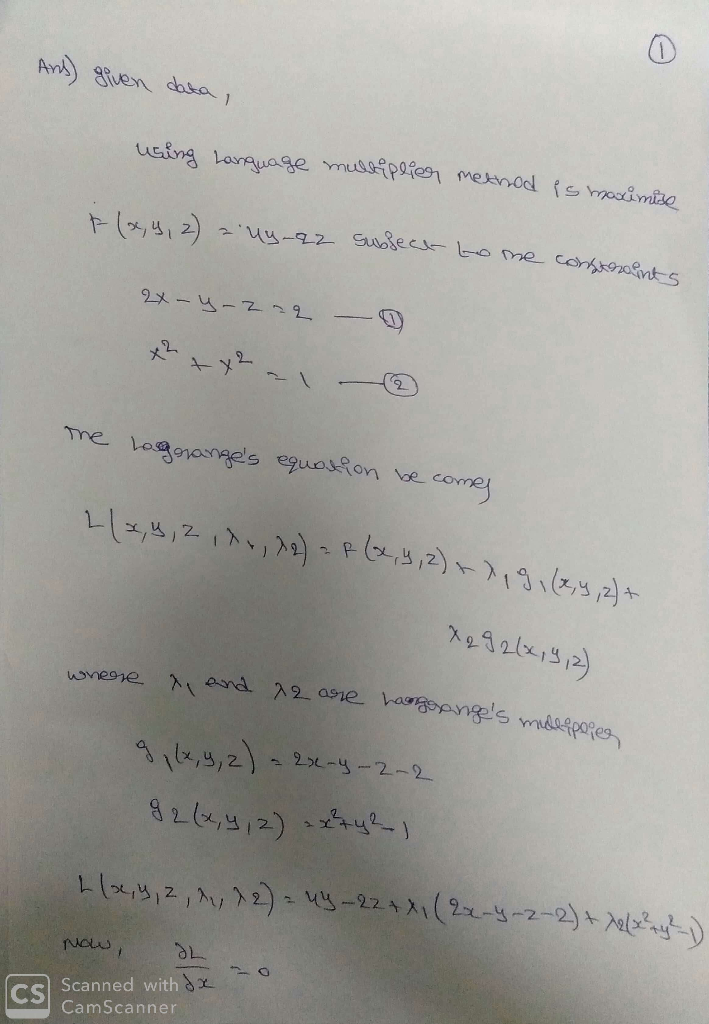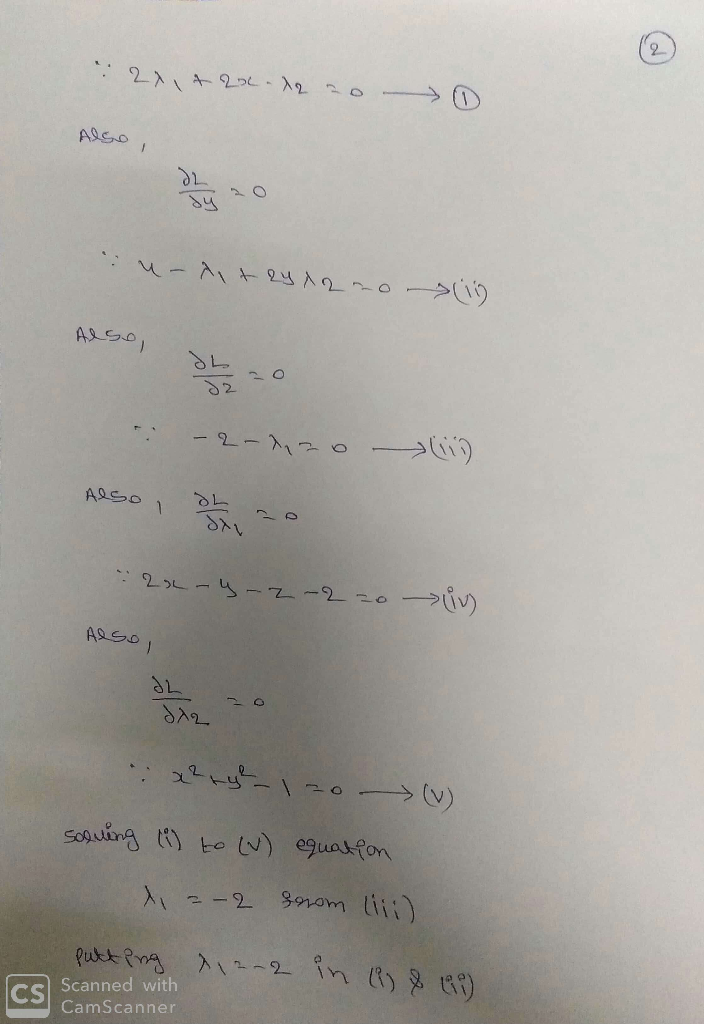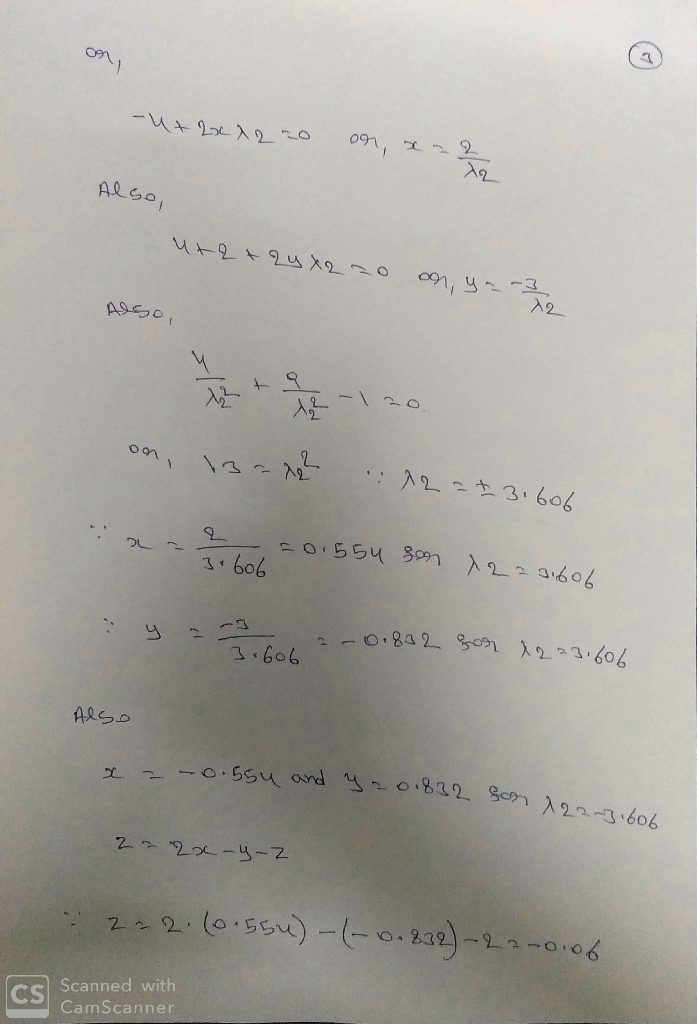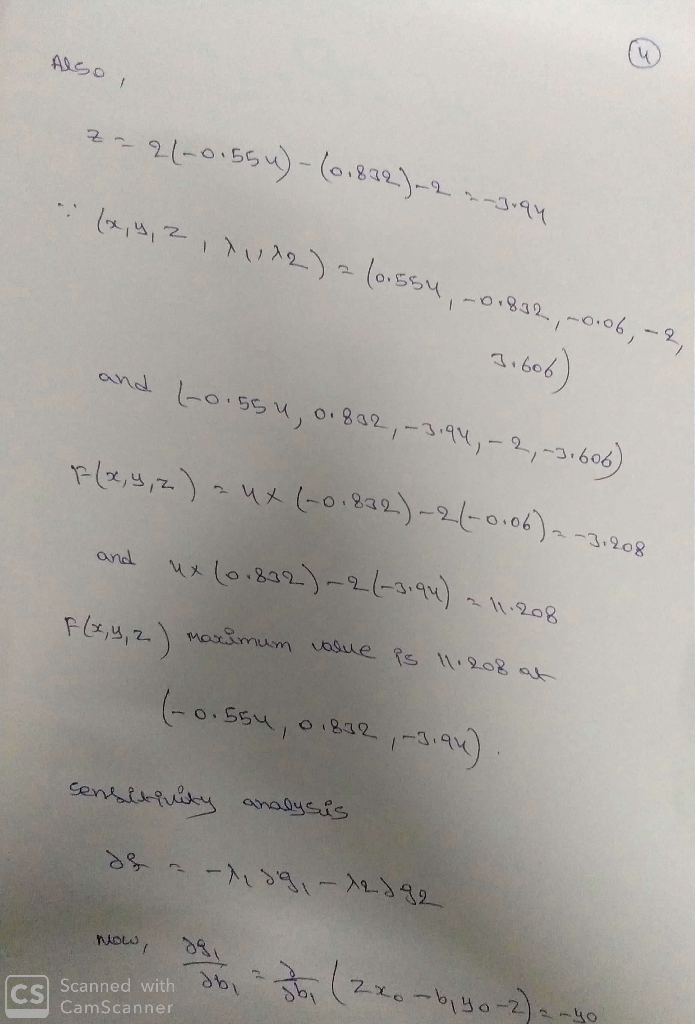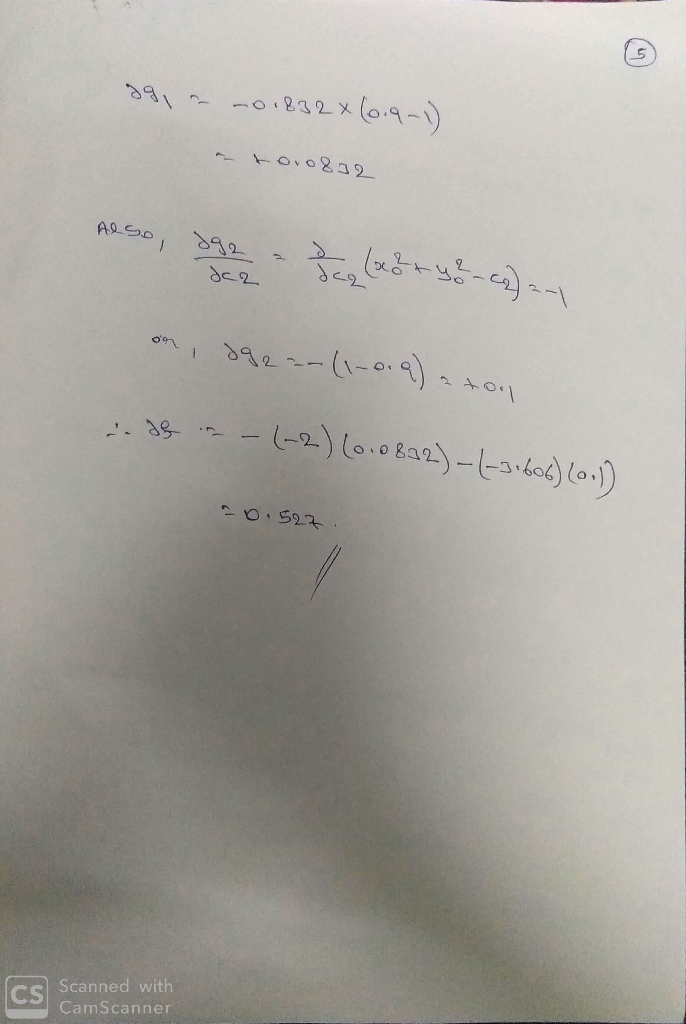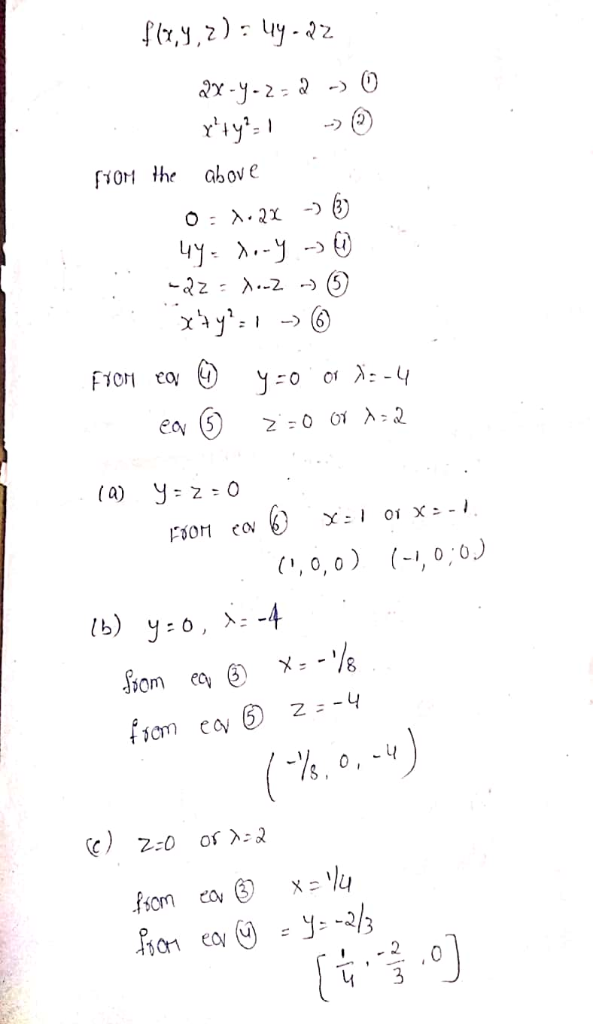​​​​​​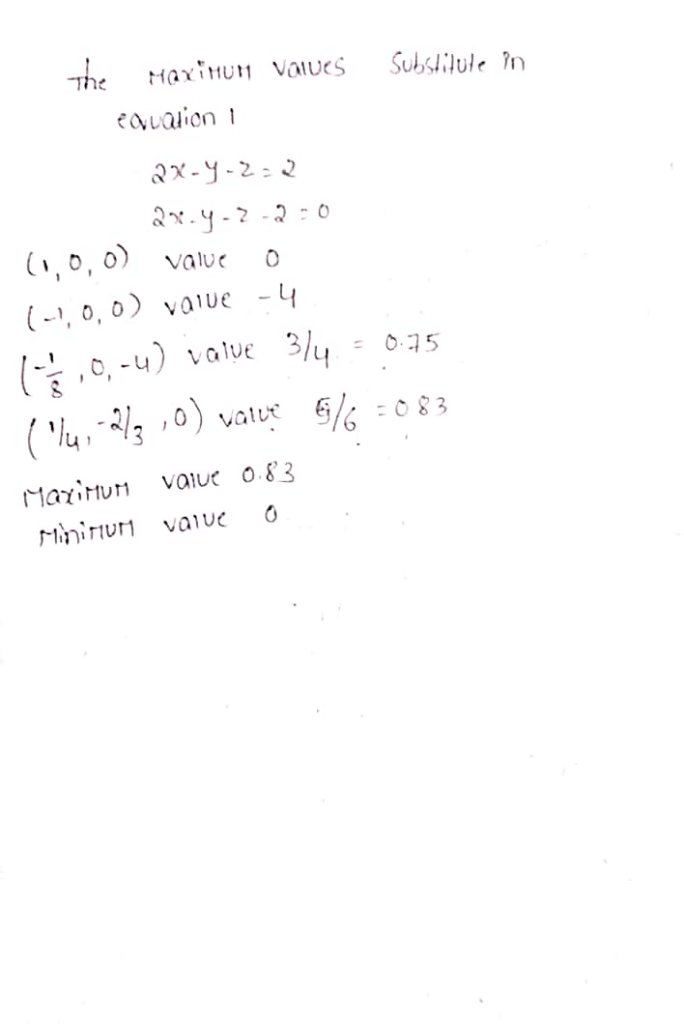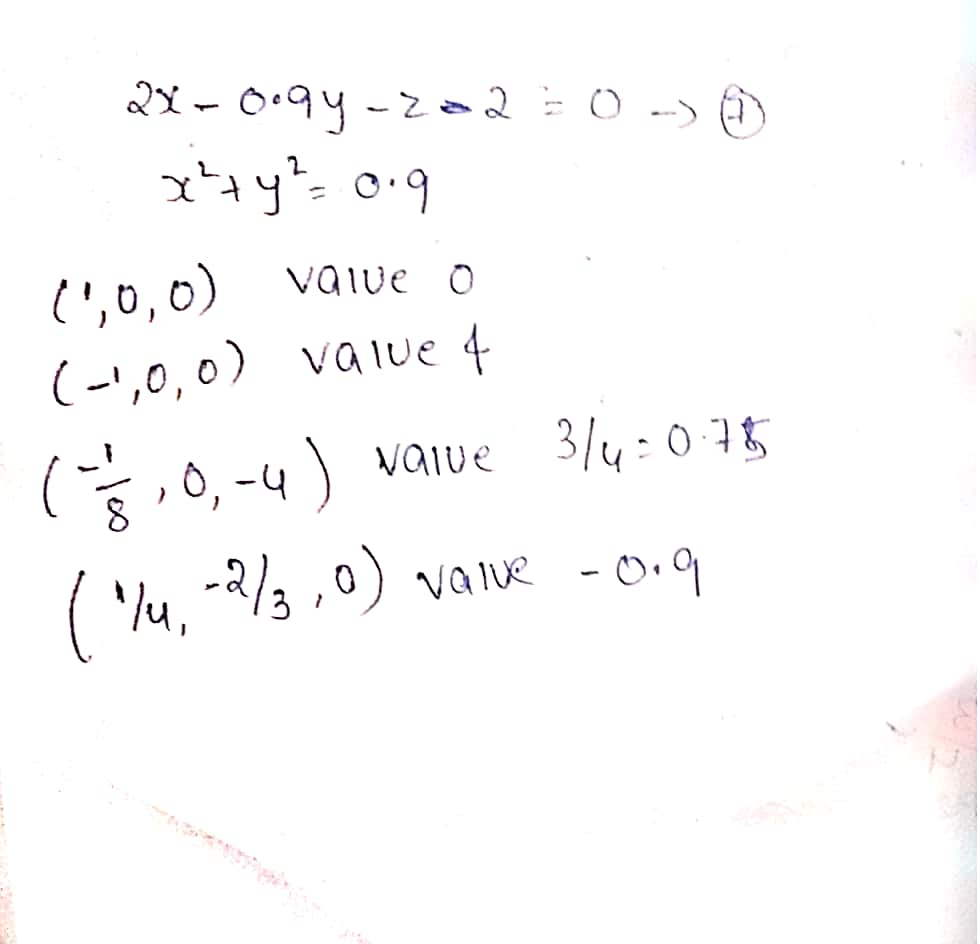#### Earn Coins

Coins can be redeemed for fabulous gifts.

Similar Homework Help Questions
• ### Solve the following problem using Lagrange multiplier method: Maximize f(x,y,z) = 4y-2z subject to the constraints...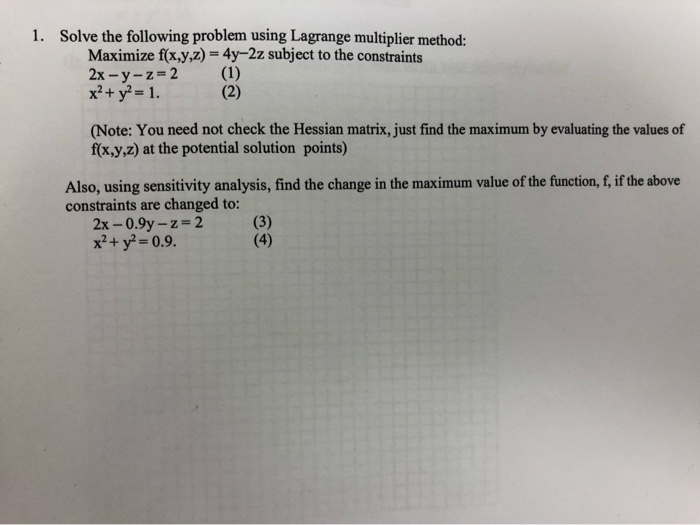Solve the following problem using Lagrange multiplier method: Maximize f(x,y,z) = 4y-2z subject to the constraints 2x-y-z 2 x2+y2- 1 1. (1) (2) (Note: You need not check the Hessian matrix, just find the maximum by evaluating the values of f(x,y,z) at the potential solution points) Also, using sensitivity analysis, find the change in the maximum value of the function, f, if the above constraints are changed to: (3) (4) 2x -0.9y-z 2 x2+ y2- 0.9. Solve the following problem...

• ### please answer step by step Solve the following problem using Lagrange multiplier method: Maximize f(x.y,z) =...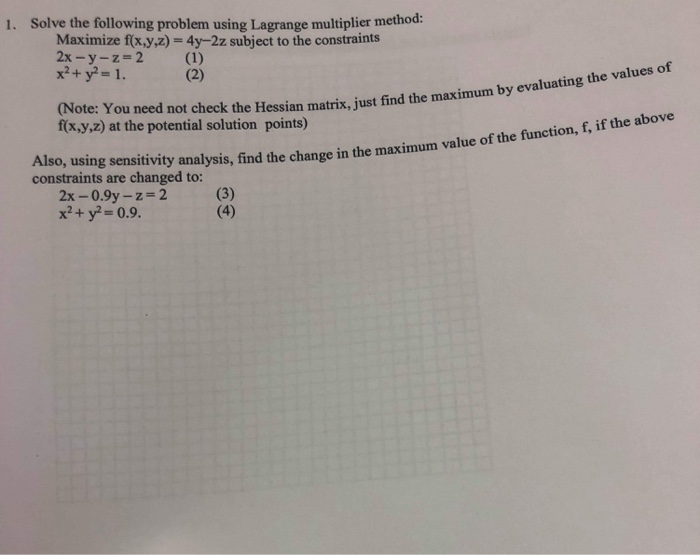please answer step by step Solve the following problem using Lagrange multiplier method: Maximize f(x.y,z) = 4y-2z subject to the constraints 2x-y-z 2 x2+ y2-1 1. (1) (2) (Note: You need not check the Hessian matrix, just find the maximum by evaluating the values of f(x,y,z) at the potential solution points) Also, using sensitivity analysis, find the change in the maximum value of the function, f, if the above changed to: (3) (4) constraints are 2x-0.9y-z 2 x2+y2-0.9. Solve the...

• ### Use Lagrange multiplier to determine the maximum and minimum values of (f,x,y,z) = x^2 +y^2 +z^2 ...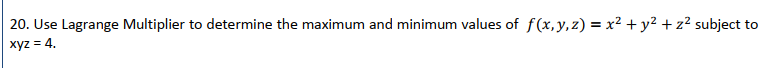Use Lagrange multiplier to determine the maximum and minimum values of (f,x,y,z) = x^2 +y^2 +z^2 subject to xyz=4 Detailed solution please. Thank you! 20. Use Lagrange Multiplier to determine the maximum and minimum values of f(x, y, z)-x2 + y2 +12 subject to 20. Use Lagrange Multiplier to determine the maximum and minimum values of f(x, y, z)-x2 + y2 +12 subject to

• ### a) Solve the following problem using Lagrange multiplier method. Minimize fCX)-x1+ x2+X 4. subject to: x2+x-3...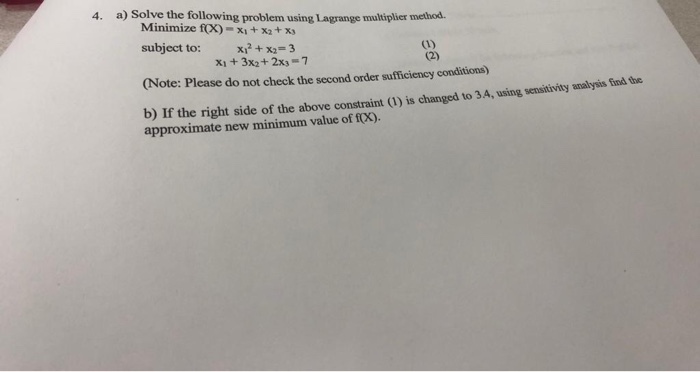a) Solve the following problem using Lagrange multiplier method. Minimize fCX)-x1+ x2+X 4. subject to: x2+x-3 X1+3x2+ 2x)- 7 (1) (2) (Note: Please do not check the second order sufficiency conditions) b) If the right side of the above constraint (1) is changed to 3.4, using sensitivity analysis find the approximate new minimum value of fX). a) Solve the following problem using Lagrange multiplier method. Minimize fCX)-x1+ x2+X 4. subject to: x2+x-3 X1+3x2+ 2x)- 7 (1) (2) (Note: Please do...

• ### Use Lagrange multipliers to find the min and max of f(x,y,z) = x2-y2+ 2z subject to the constraint x2 + y2 + z2 = 1.

Use Lagrange multipliers to find the min and max of f(x,y,z) = x2-y2+ 2z subject to the constraint x2 + y2 + z2 = 1.

• ### Use the method of Lagrange multipliers to find the extreme value of the function f(x, y,...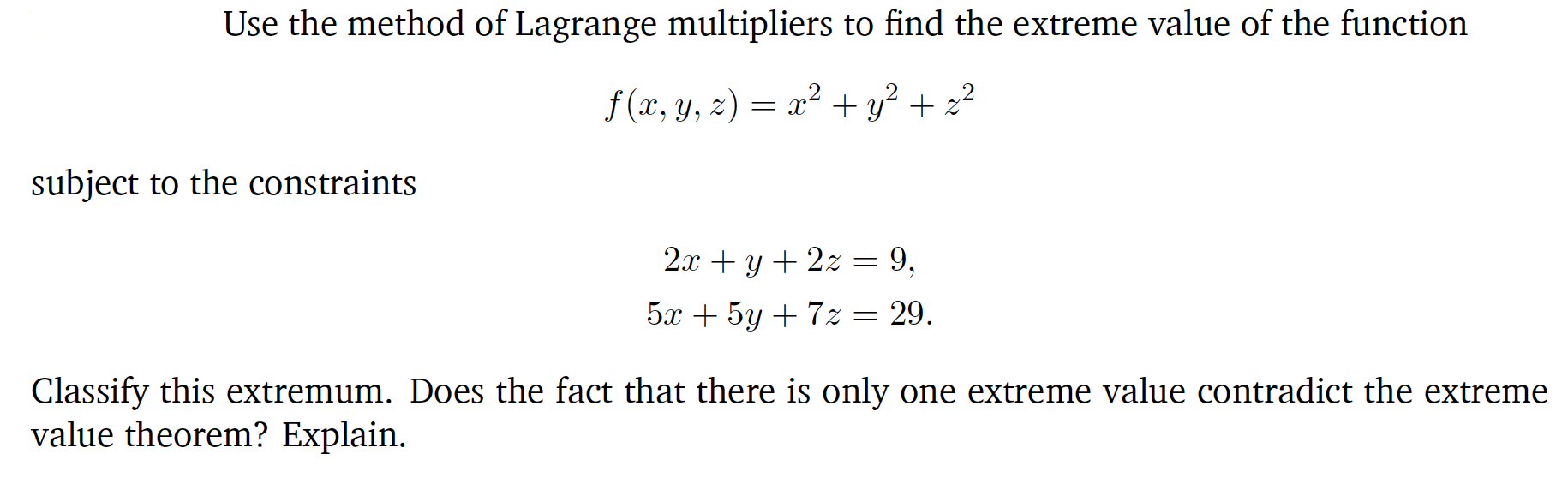Use the method of Lagrange multipliers to find the extreme value of the function f(x, y, z) = x2 + y2 + 22 subject to the constraints 2x + y + 2z = 9, 5x + 5y + 72 = 29. Classify this extremum. Does the fact that there is only one extreme value contradict the extreme value theorem? Explain.

• ### Find the values of x2 0 and y 2 0 that maximize z 10x+ 12y, subject to each of the following sets of constraints (a) x...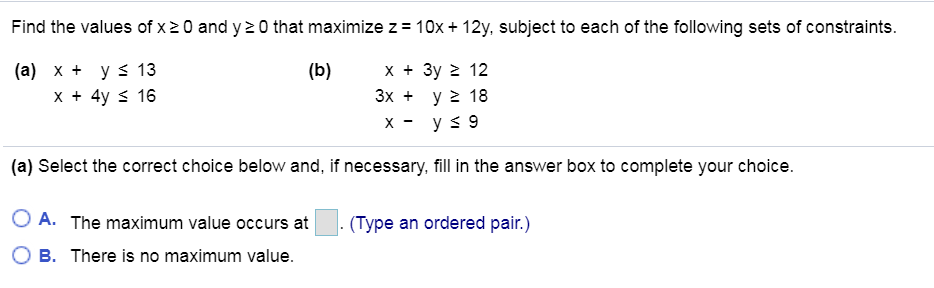Find the values of x2 0 and y 2 0 that maximize z 10x+ 12y, subject to each of the following sets of constraints (a) x ys 13 x +4y s 16 (b) x 3y 2 12 3x y2 18 (a) Select the correct choice below and, if necessary, fill in the answer box to complete your choice. OA. The maximum value occurs at .(Type an ordered pair.) 0 B. There is no maximum value. Find the values of x2...

• ### Solve the following problems by USING Lagrange multipliers. (a) Find the maximum and minimum values of f(x, y, z) = x^2...

Solve the following problems by USING Lagrange multipliers. (a) Find the maximum and minimum values of f(x, y, z) = x^2 + y^2 + z^2 subject to the constraint (x − 1)^2 + (y − 2)^2 + (z − 3)^2 = 4 (b) Find the maximum and minimum values of f(x, y, z) = x^2 + y^2 + z^2 subject to the constraints (x − 1)^2 + (y − 2)^2 + (z − 3)^2 = 9 and x − 2z...

• ### Use Lagrange multipliers to find both the maximum and minimum values of f(z, y, z) = 2x + y-2z on...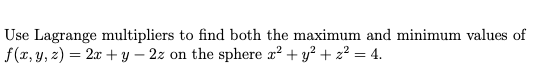Can you help me? This is calculus 3. Use Lagrange multipliers to find both the maximum and minimum values of f(z, y, z) = 2x + y-2z on the sphere r2 + y2 + z2-4. Use Lagrange multipliers to find both the maximum and minimum values of f(z, y, z) = 2x + y-2z on the sphere r2 + y2 + z2-4.

• ### Using the method of Lagrange Multipliers, the extrema of f(x,y) = x +y subject to the...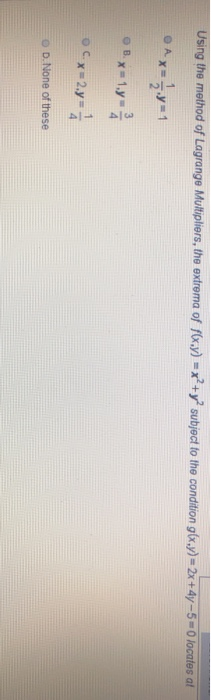Using the method of Lagrange Multipliers, the extrema of f(x,y) = x +y subject to the condition g(x,y) = 2x+4y -5 - O locates at B.x=1. 2 O x =2.y=0 OD. None of these The extrema of f(x,y) = x + y2 - 4x -6y +17, at critical point (2,3) is A. Maxima NB Minima O C. Saddle Point D. None of these